# Reaction Coordinates The Hammond Postulate Energy transition state

• Slides: 12Reaction Coordinates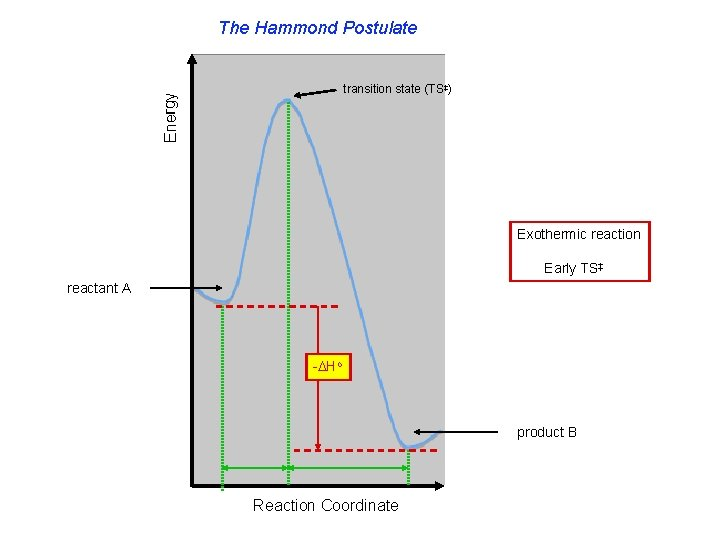The Hammond Postulate Energy transition state (TS‡) Exothermic reaction Early TS‡ reactant A - Ho product B Reaction Coordinate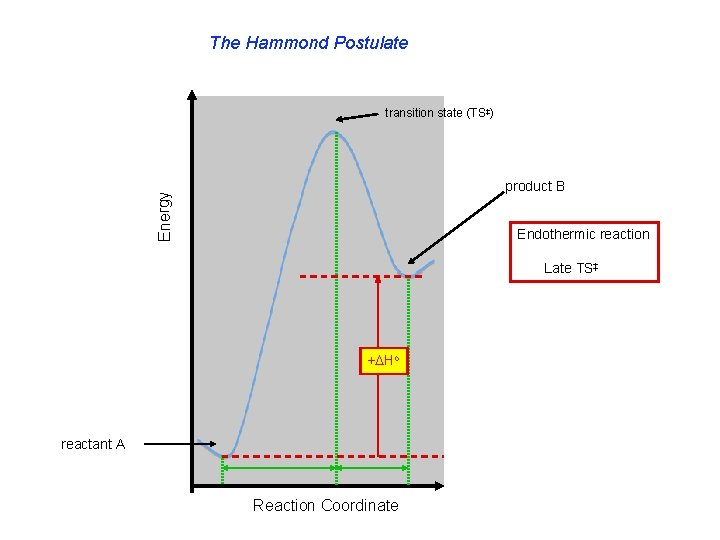The Hammond Postulate transition state (TS‡) Energy product B Endothermic reaction Late TS‡ + Ho reactant A Reaction Coordinate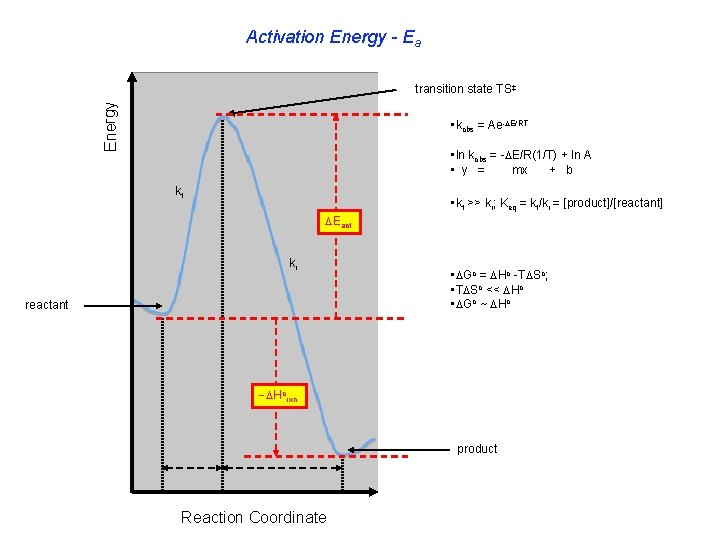Activation Energy - Ea Energy transition state TS‡ • kobs = Ae- E/RT • ln kobs = - E/R(1/T) + ln A • y = mx + b kf • kf >> kr; Keq = kf/kr = [product]/[reactant] Eact kr reactant • Go = Ho -T So; • T So << Ho • Go ~ Ho Horxn product Reaction Coordinate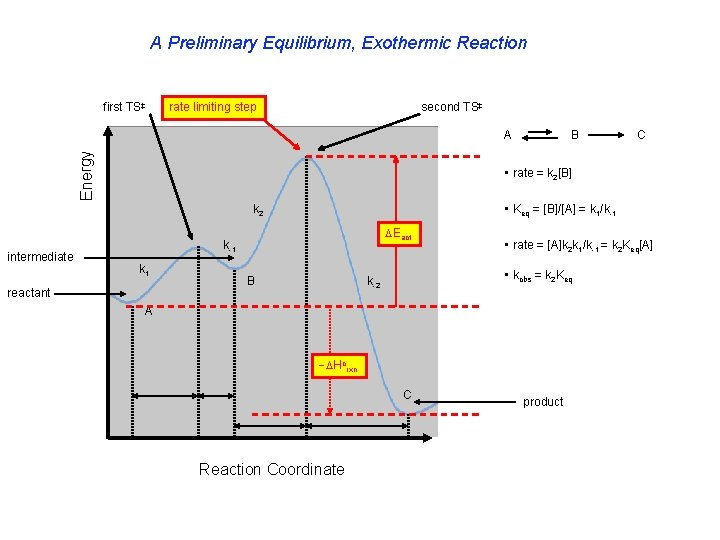A Preliminary Equilibrium, Exothermic Reaction first TS‡ rate limiting step second TS‡ Energy A C • rate = k 2[B] • Keq = [B]/[A] = k 1/k-1 k 2 intermediate B Eact k-1 k 1 reactant B • rate = [A]k 2 k 1/k-1 = k 2 Keq[A] • kobs = k 2 Keq k-2 A Horxn C Reaction Coordinate product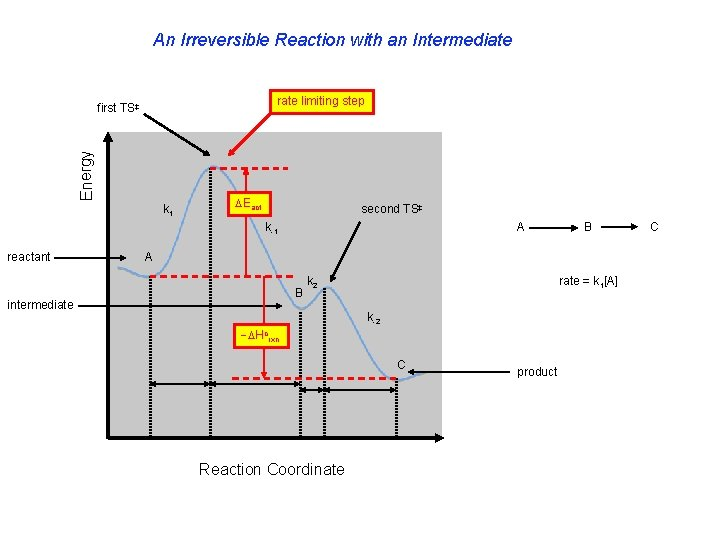An Irreversible Reaction with an Intermediate rate limiting step Energy first TS‡ k 1 Eact second TS‡ k-1 reactant A B intermediate k 2 rate = k 1[A] k-2 Horxn C Reaction Coordinate product CEnergy Competitive Irreversible Reactions - I kgreen Eact < > kblue Eact < > kred Eact reactant A Hogreen > product B product C product D Reaction Coordinate Hoblue > Hored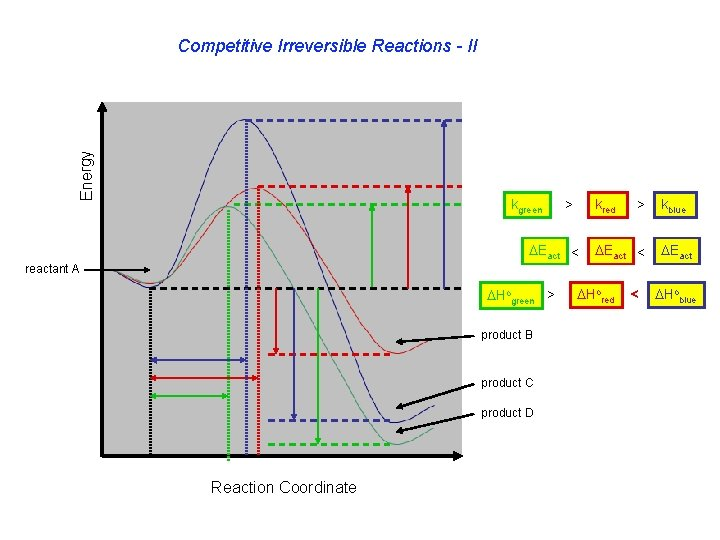Energy Competitive Irreversible Reactions - II kgreen > kred Eact < > Eact < kblue Eact reactant A Hogreen > product B product C product D Reaction Coordinate Hored < Hoblue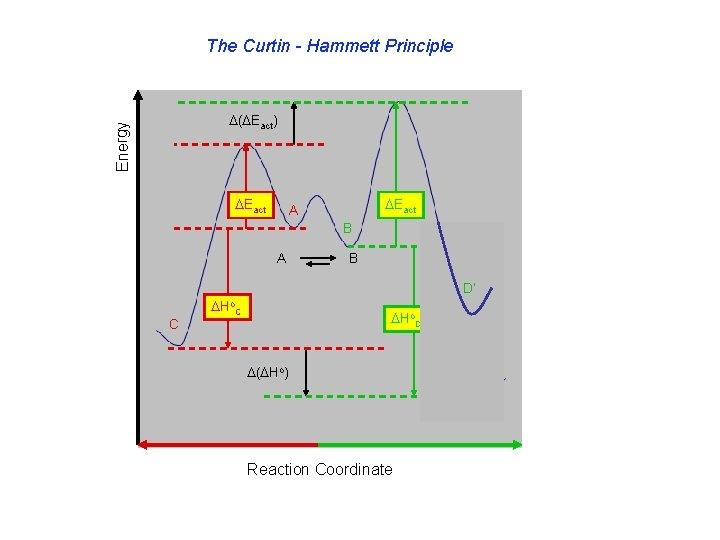The Curtin - Hammett Principle Energy ( Eact) Eact A B D’ C Ho. D ( Ho) Reaction Coordinate D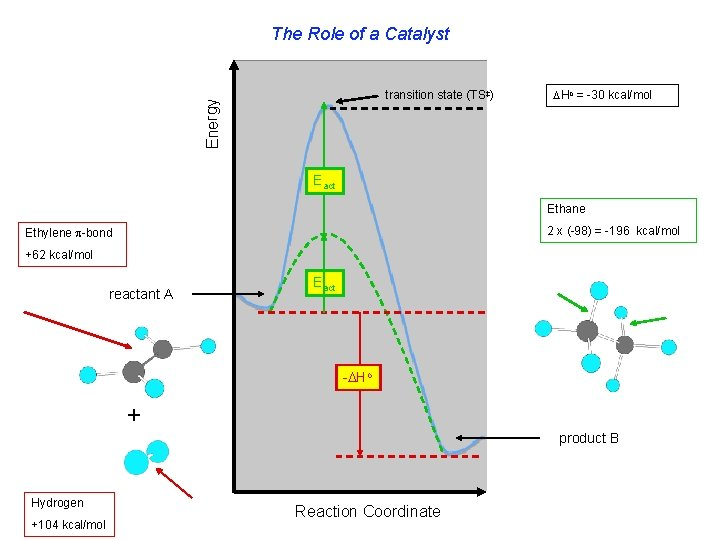The Role of a Catalyst Energy transition state (TS‡) Ho = -30 kcal/mol Eact Ethane 2 x (-98) = -196 kcal/mol Ethylene -bond +62 kcal/mol reactant A Eact - Ho + product B Hydrogen +104 kcal/mol Reaction CoordinateThe Distribution of Reactive Molecules Fraction of molecules with energy E T 1 T 4 > T 3 > T 2 T 3 T 4 Energy T 1The End F. E. Ziegler 2009